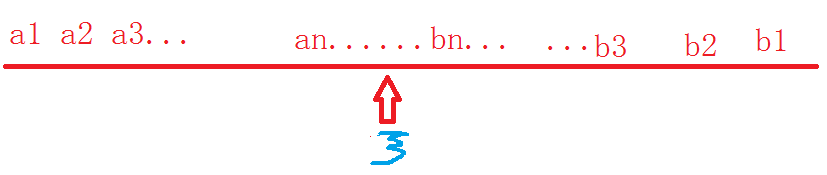# 6 theorems relating to real number's comleteness

note:all the 6 theorems are applicable only over real number field, other than rational umber. cause they are
incorrect in it

NO.1 theorem of closed nested intervals

DEFINITION of "CLOSED NESTED INTERVAL"
suppose the series of closed intervals as ({[a_{n},b_{n}]}) has such qulities as below:
(i)([a_{n},b_{n}]supset [a_{n+1},b_{n+1}],n=1,2,3cdotcdotcdot)
(ii)(lim_{n oinfty}(b_{n}-a_{n})=0)
we call ({[a_{n},b_{n}]}) "a closed nested interval".from the chart above, we can infer that:

theorem of "closed nested intervals":
if %{[a_{n},b_{n}]}% is a closed nested intervals, then there is only one point (xi), with (xiin[a_{n},b_{n}]),n=1,2,3,...
ie: (a_{n}leqslant xi ,n=1,2,cdotcdotcdot.)

prove:

## 随便看看

### Our take on Derby vs. Meteor

Our take on Derby vs. Meteor - Derby blog Our take on Derby vs. Meteor Apr 14th, 2012 We have received a number of requests for a comparison. I’d like to thank Nick Retallack, w...